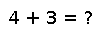# significand

significand(x)

Extract the `significand(s)` (a.k.a. mantissa), in binary representation, of a floating-point number or array. If `x` is a non-zero finite number, than the result will be a number of the same type on the interval \$[1,2)\$. Otherwise `x` is returned.

``````julia> significand(15.2)/15.2
0.125

julia> significand(15.2)*8
15.2``````

## Examples

Checking you are not a robot: